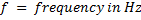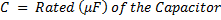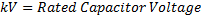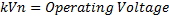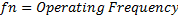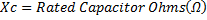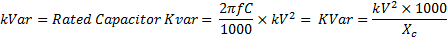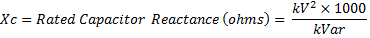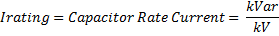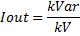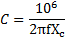﻿ NEPSI - Capacitive Kvar Calculator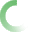The fields with the red border are required.# CALCULATION OF CAPACITOR KVAR

The calculators on this web page are used to calculate kvar and other capacitor parameters for single-phase capacitors commonly used on medium voltage capacitor banks and harmonic filter banks. Use calculator-1 when capacitor voltage, capacitance, and frequency are known. Use calculator-2 when capacitor voltage, capacitive reactance, and frequency are known.

Also on this page are calculators (calculator-3 and calculator-4) for applying capacitors on systems that deviate from their nameplate rating. Use these calculators to determine resulting output kvar and current for your specific application.

Calculator-1

Known variables: Capacitor Frequency, Capacitor Voltage and Capacitance

Calculator-2

Known variables: Capacitor Frequency, Capacitor Voltage, and Reactance

 Capacitor rated frequency (Hz): Capacitor rated frequency (Hz): Capacitor rated Voltage (kV): Capacitor rated Voltage (kV): Calculated rated Capacitance (uF): Capacitor Reactance (Ohms): Capacitor kVar: Capacitor kVar: Capacitor Reactance (Ohms): Capacitor Current Rating (Amps): Capacitor Current Rating: Calculated Capacitance (uF):

For application of the above rated capacitors on systems that deviates from their nameplate value, use the calculators below. Calculator-3 uses calculator-1 input values. Calculator-4 uses calculator-2 input values.

Calculator-3

Known variables: Capacitor Voltage and Capacitor Frequency

Calculator-4

Known variables: Capacitor Voltage and Capacitor Frequency

 Operating Voltage (kV): Operating Voltage (kV): Operating Frequency (Hz): Operating Frequency (Hz): Output Kvar: Output Kvar: Output Current (Amps): Output Current (Amps): "" ""

Variables